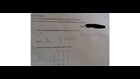Breaking News
More () »

# People are losing it over these ‘incorrect’ answers on a math test

Are the answers wrong?(Imgr)

Louisville, Ky. (WHAS11) – A photo posted to Imgur has people on the Internet up in arms.

The picture is of a math test given to an elementary school student. The question on the test asks, “What is 5 x 3?” and it also asks that the student show his/her work using the “repeated addition strategy.”

The student wrote 5+5+5, but the teacher points out should be 3+3+3+3+3.

Many people commenting on the image side with the student and believe the answer is correct.

Other people point out that, technically, the equation reads 5 times of 3. To put that in mathematical terms, it would be like thinking of it as 5y. 5y = 5 times y = y+y+y+y+y. So, if y = 3, then 5(3) = 5 times 3 = 3+3+3+3+3.

One thing we can all agree on is: Math is hard.